# Markov Duality

markov_processes duality

Definition. Markov processes $$X$$ and $$Y$$ are dual with respect to function $$H$$ if

$\expe_{X_0 = x} H(X_t, y) = \expe_{Y_0 = y} H(x, Y_t).$

When $$X$$ and $$Y$$ have generator $$L^X$$ and $$L^Y$$, this is equivalent to

$L^X H(x, y) = L^Y H(x, y).$

This equivalence can be seen by taking $$\partial_t|_{t = 0}$$ and noting the initial condition is tautology ($$H(x, y) = H(x, y)$$).

We derive the duality between $$q$$-TASEP and $$q$$-TAZRP, and between ASEP occupation and location processes. These results can be found in e.g. [{borodin-corwin-sasamoto14}].

## duality between $$q$$-TASEP and $$q$$-TAZRP

qTASEP qTAZRP duality

Let $$X$$ be $$q$$-TASEP with $$N$$ particles, and $$Y$$ be $$q$$-TAZRP with $$N$$ sites. More specifically, $$X_k$$ is the location of $$k$$th particle of $$q$$-TASEP and $$Y_k$$ is the number of particles at site $$k$$. Let $$a_i$$ be the rate of jumps by the $$i$$th particle in $$q$$-TASEP, and of from site $$i$$ to site $$i - 1$$ in $$q$$-TAZRP.

The generator of $$q$$-TASEP is

$L^X f(x) = \sum_i a_i (1 - q^{x_{i - 1} - x_i - 1}) (f(x + e_i) - f(x))$

and that of $$q$$-TAZRP is

$L^Y f(x) = \sum_i a_i (1 - q^{y_i}) (f(y - e_i + e_{i - 1}) - f(y))$

Let

$H(x, y) = \prod_{i = 1 : N} q^{(x_i + i) y_i}.$

Claim. $$X$$ and $$Y$$ are dual w.r.t. $$H$$.

Proof.

$L^X H(x, y) = \sum_i a_i (1 - q^{x_{i - 1} - x_i - 1}) (q^{y_i} - 1) H(x, y) = L^Y (x, y).$

$$\square$$.

## duality between ASEP occupation and location processes

ASEP duality

Let $$\eta$$ be the occupation process of ASEP with rate $$p$$ to the right and $$q$$ to the left:

$L^\eta f(\eta) = \sum_i p \eta_i (1 - \eta_{i + 1}) (f(\eta - e_i + e_{i + 1}) - f(\eta)) + q (1 - \eta_i) \eta_{i + 1} (f(\eta - e_{i + 1} + e_i) - f(\eta)).$

and $$X$$ be the location process of ASEP with rate $$p$$ to the left and $$q$$ to the right:

$L^X f(x) = \sum_{i \in l(x)} p (f(x - e_i) - f(x)) + \sum_{i \in r(x)} q (f(x + e_i) - f(x)),$

where $$l(x) = \{i : x_{i - 1} + 1 < x_i\}$$ and $$r(x) = \{i : x_i + 1 < x_{i + 1}\}$$ are the left and right edges of clusters.

Let

\begin{align} \tau &= p / q\\ N_x (\eta) &= \sum_{i \le x} \eta_i\\ H(\eta, x) &= \prod_i \tau^{N_{x_i} (\eta)} \qquad (1) \\ G(\eta, x) &= \prod_i \tau^{N_{x_i - 1} (\eta)} \eta_{x_i} \end{align}

Claim 1. $$X$$ and $$\eta$$ are dual w.r.t. both $$H$$ and $$G$$.

Proof. Let us consider $$G$$ first. We consider one $$x$$-cluster $$x_{l : r}$$. Locally, there are 5 cases when at least one of the summands in $$L^\eta G$$ or $$L^X G$$ is nonzero. We use crosses on the horizontal axis to denote the $$x$$-particles, and lines to denote the profile of $$\eta$$. That is, $$\eta = 0$$ wherever the line touches the horizontal axis and $$\eta = 1$$ wherever the line is above the horizontal axis. Here are the 5 cases.

• Case 1: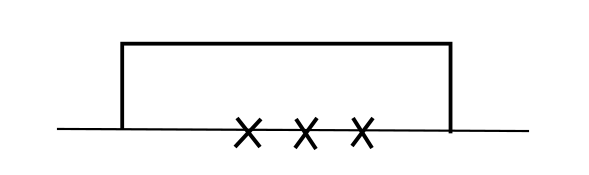• Case 2: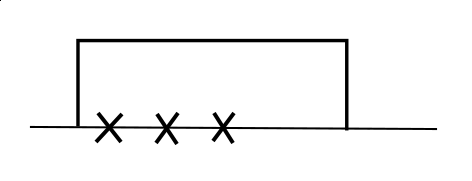• Case 3: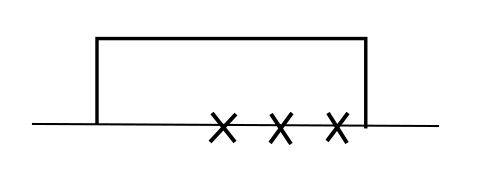• Case 4: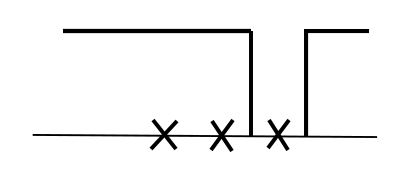• Case 5: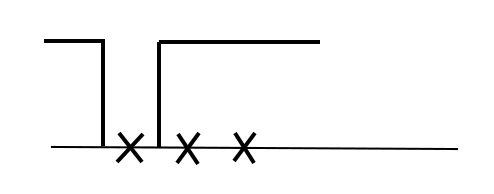In case 1 2 and 3 $$x_{l : r}$$ is fully covered by $$\eta$$. $$G(x, \eta)$$ is locally nonzero, but when $$\eta$$ is altered slightly, in case 2 and 3 $$G$$ may be zero.

In case 4 (resp. 5) all but $$x_r$$ (resp. $$x_l$$) is covered. $$G(x, \eta)$$ is zero, but when $$\eta$$ or $$x$$ is altered slightly, $$G$$ may become locally nonzero.

It suffices to consider one $$x$$-cluster $$x_{l : r}$$.

In case 1:

$L^\eta G(\eta, x) = 0$

Consider $$L^X G(\eta, x)$$.

• The term corresponding to $$x_l$$ jumping to the left is $$p G(\eta, x) (\tau^{-1} - 1) = (q - p) G(\eta, x)$$. This will also appear in Case 3.
• The term corresponding to $$x_r$$ jumping to the right is $$q G(\eta, x) (\tau - 1) = (p - q) G(\eta, x)$$. This will also appear in Case 2.

Therefore

$L^X G(\eta, x) = (q - p) G(\eta, x) + (p - q) G(\eta, x) = 0.$

In case 2:

\begin{align} L^\eta G(\eta, x) &= - q G(\eta, x)\\ L^X G(\eta, x) &= - p G(\eta, x) + (p - q) G(\eta, x) = - q G(\eta, x). \end{align}

Similarly in Case 3:

\begin{align} L^\eta G(\eta, x) &= - p G(\eta, x) = L^x G(\eta, x)\\ \end{align}

In case 4:

$L^\eta G(\eta, x) = q G(\eta - e_{x_r + 1} + e_{x_r}, x) = q G(\eta, x + e_r) = L^X G(\eta, x)$

In case 5:

$L^\eta G(\eta, x) = p G(\eta - e_{x_l - 1} + e_{x_l}) = p G(\eta, x - e_l) = L^X G(\eta, x).$

This concludes the proof that $$G$$ is a duality function. Now let us turn to $$H$$. In terms of $$L^\eta$$ there are two cases where $$H(x, \eta)$$ changes and has nonzero corresponding summands:

• E1. For some $$x_i$$ in the $$X$$-process, $$\eta_{x_i} = 0$$, $$\eta_{x_i + 1} = 1$$. In other words, there is an $$X$$-particle just to the left of the left edge of an $$\eta$$-cluster. Then the $$\eta$$-particle jumps to the left.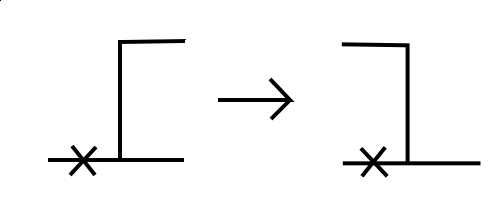• E2. For some $$x_i$$ in the $$X$$-process, $$\eta_{x_i} = 1$$, $$\eta_{x_i + 1} = 0$$. In other words, there is an $$X$$-particle on the right edge of an $$\eta$$-cluster. Then the $$\eta$$-particle jumps to the right.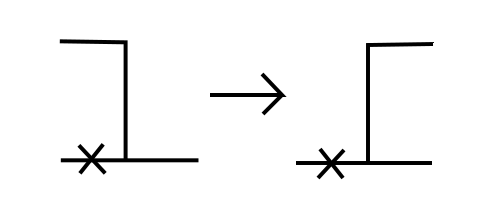The summands are respectively

\begin{align} q (\tau - 1) H(x, \eta) &= (p - q) H(x, \eta) \\ p (\tau^{-1} - 1) H(x, \eta) &= (q - p) H(x, \eta) \end{align}

In terms of $$L^X$$ there are also two cases, which are in a sense dual to the $$L^\eta$$. We use circles to denote empty site in the $$X$$-process.

• X1. For some site $$x$$ in the $$\eta$$-process, $$\eta_x = 1$$, there is an $$x_i = x - 1$$ of the $$X$$-process, but no $$X$$-particle at $$x$$. In other words, there is an $$\eta$$-particle just to the right of the right edge of an $$X$$-cluster. Then the $$x_i$$ particle jumps to the right.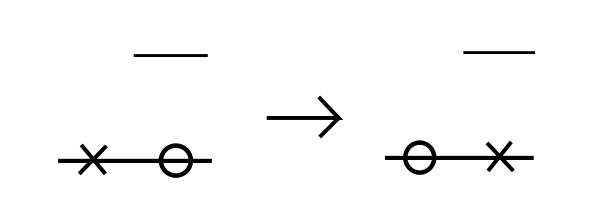• X2. For some site $$x$$ in the $$\eta$$-process, $$\eta_x = 1$$, there is an $$x_i = x$$ of the $$X$$-process, but no $$X$$-particle at $$x - 1$$. In other words, there is an $$\eta$$-particle on the left edge of an $$X$$-cluster. Then the $$x_i$$ particle jumps to the left.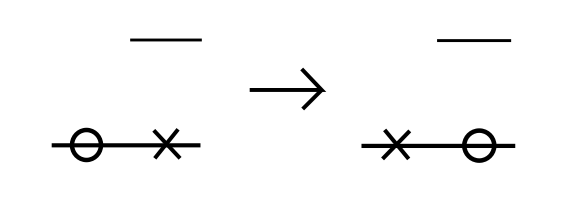These two cases also correspond to

\begin{align} (p - q) H(x, \eta) \\ (q - p) H(x, \eta) \end{align}

respectively.

Now consider one $$X$$-cluster. We can pair the two cases $$E_1$$ and $$E_2$$ in $$L^\eta$$ and removing the corresponding portions (since the contribution of $$E_1$$ and $$E_2$$ cancels each other) to reduce the configuration without $$\eta$$-"valleys" or -"hills".

For example here is such a pairing, and everything in the red frame can be removed, reducing the configuration to case 3 in the following.we are left with verifying the duality in the following 4 cases wihtout "valleys" or "mountains":

• case 1: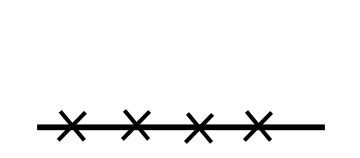• case 2: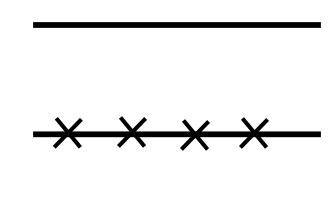• case 3: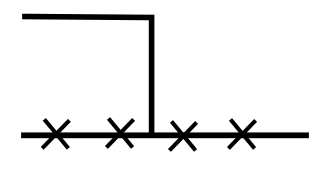• case 4: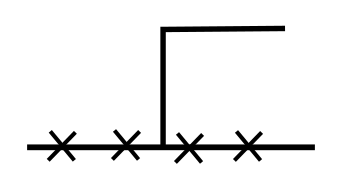All of them are easily verified:

• In case 1 and 2, $$L^\eta H = L^x H = 0$$.
• In case 3, $$L^\eta H = L^x H = (q - p) H$$.
• In case 4, $$L^\eta H = L^x H = (p - q) H$$.

$$\square$$

Remark. Note that duality does not hold in the ASEP case if, as in the $$q$$TASEP case we introduce the bond jump rate parameters $$a_i$$. More specifically, duality does not hold for the following $$L^\eta$$, $$L^X$$, and $$G$$ as in (1):

\begin{align} L^\eta f(\eta) &= \sum_i a_i p \eta_i (1 - \eta_{i + 1}) (f(\eta - e_i + e_{i + 1}) - f(\eta)) + a_i q (1 - \eta_i) \eta_{i + 1} (f(\eta - e_{i + 1} + e_i) - f(\eta))\\ L^X f(x) &= \sum_{i \in l(x)} a_{x_i - 1} p (f(x - e_i) - f(x)) + \sum_{i \in r(x)} a_{x_i} q (f(x + e_i) - f(x)) \end{align}

That is, for the $$x_i$$ particle in the $$X$$-process, the rate of jumping to the left is $$a_{x_i - 1} p$$ and to the right is $$a_{x_i} q$$; and for the $$\eta$$-process at site $$x$$, the rate of an unobstructed particle jumping to the left is $$a_{x - 1} q$$ and to the right $$a_x p$$. To see that $$G$$ is not a duality function, for example consider Case 2 in the proof of $$G$$ being the duality function in Claim 1.

\begin{align} L^\eta G(\eta, x) = - q a_{x_{l} - 1} G(\eta, x)\\ L^X G(\eta, x) = (- p a_{x_l - 1} + (p - q) a_{x_r}) G(\eta, x). \end{align}

They are not equal unless $$p = q$$ or all the $$a_i$$s are equal. In fact if either of these conditions is satisfied, $$G$$ is a duality function.

## References

• [borodin-corwin-sasamoto14] From duality to determinants for q-TASEP and ASEP, , Ann. Probab., Vol. 42, No. 6, p.2314–2382, 11 2014. [ link ]